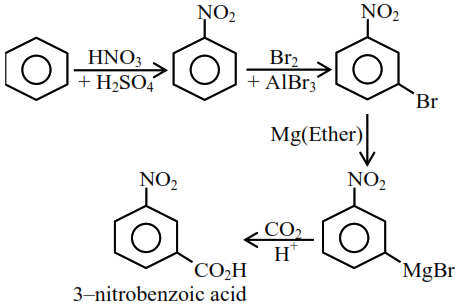Question:

The correct sequential addition of reagents in the preparation of 3-nitrobenzoic acid from benzene is:

1. $\mathrm{Br}_{2} / \mathrm{AlBr}_{3}, \mathrm{HNO}_{3} / \mathrm{H}_{2} \mathrm{SO}_{4}, \mathrm{Mg} / \mathrm{ether}, \mathrm{CO}_{2}, \mathrm{H}_{3} \mathrm{O}^{+}$

2. $\mathrm{Br}_{2} / \mathrm{AlBr}_{3}, \mathrm{NaCN}, \mathrm{H}_{3} \mathrm{O}^{+}, \mathrm{HNO}_{3} / \mathrm{H}_{2} \mathrm{SO}_{4}$

3. $\mathrm{Br}_{2} / \mathrm{AlBr}_{3}, \mathrm{HNO}_{3} / \mathrm{H}_{2} \mathrm{SO}_{4}, \mathrm{NaCN}, \mathrm{H}_{3} \mathrm{O}^{+}$

4. $\mathrm{HNO}_{3} / \mathrm{H}_{2} \mathrm{SO}_{4}, \mathrm{Br}_{2} / \mathrm{AlBr}_{3}, \mathrm{Mg} /$ ether, $\mathrm{CO}_{2}, \mathrm{H}_{3} \mathrm{O}^{+}$

Correct Option: , 4

Solution: